# Two Degree-of-Freedom PID Control for Setpoint Tracking

This example shows how to regulate the speed of an electric motor using two degree-of-freedom PID control with set-point weighting. This model uses the PID Controller (2DOF) block. The model changes the setpoint values between 60 and 30 rpm. To convert the units to rad/s for use in the PID controller, the model uses a Signal Conversion block.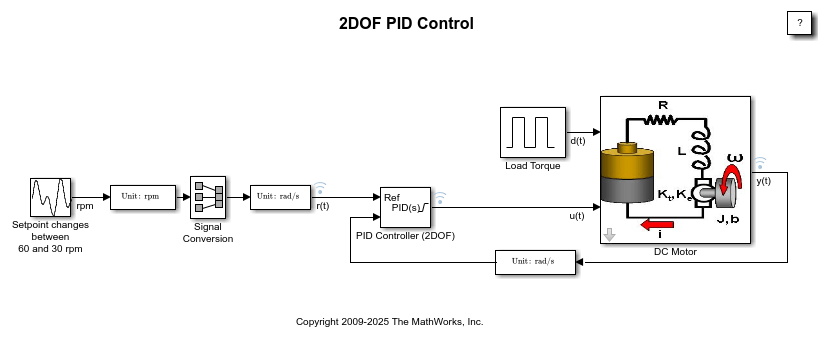The electric motor is an armature-controlled DC motor. Voltage input controls the shaft speed of the motor. The motor experiences a load torque(0-0.1 Nm).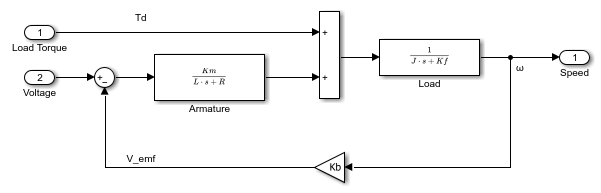### Two Degree-of-Freedom PID Control

In contrast to the PID Controller block, the PID Controller (2DOF) block provides an extra degree of freedom, allowing you to weight the setpoint as it passes through the proportional action channel and the derivative action channel.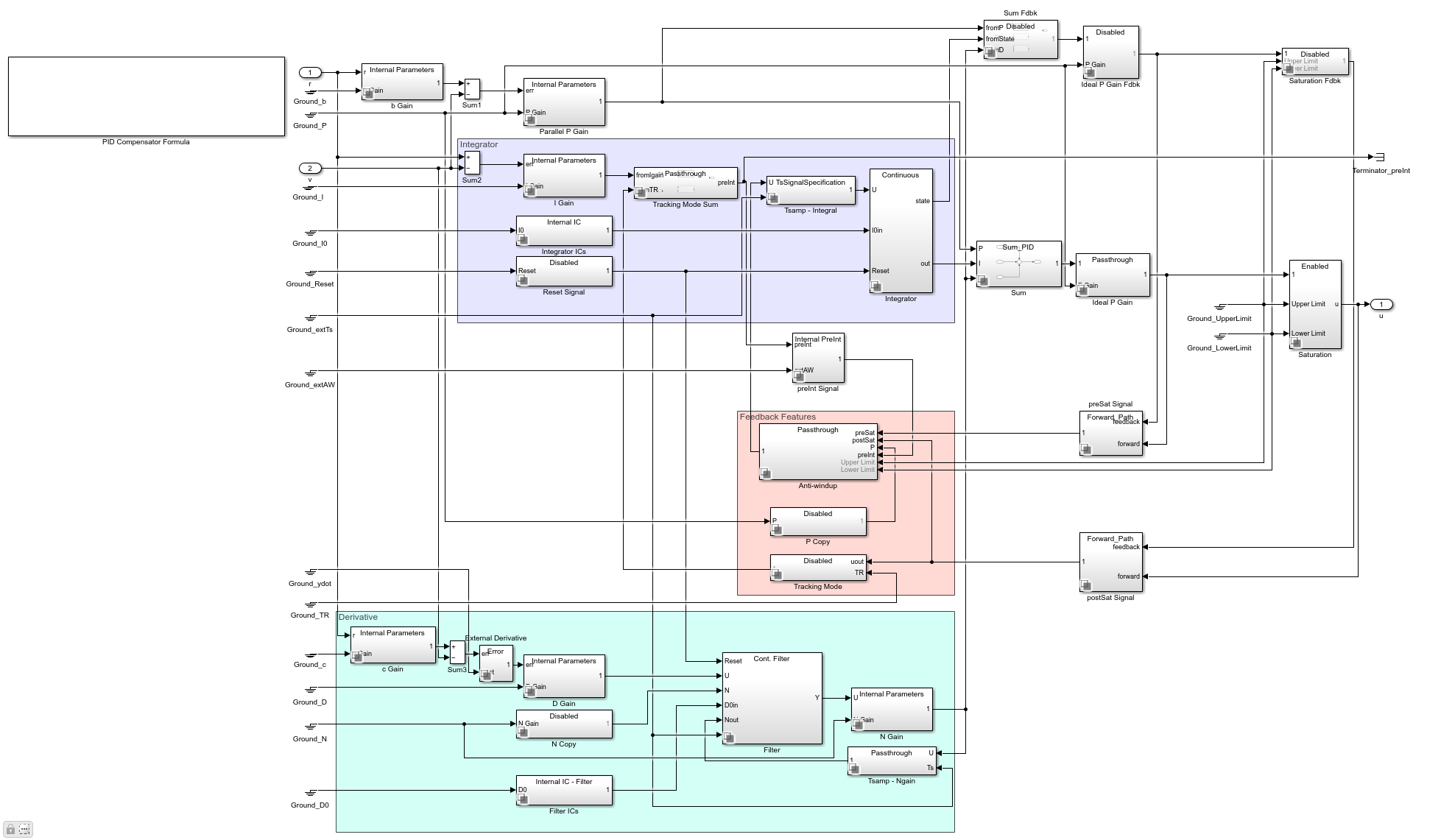The proportional action error signal is given by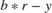.

The signal seen by the derivative action signal is given by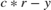,

and the integral action signal is given by.

In general, the setpoint weight c is set to 0. This value prevents undesirable transients if the setpoint changes, an effect known as derivative kick. The setpoint b affects the overshoot performance of the controller. Generally, a small b value reduces overshoot. However, smaller b values can also result in slower response to setpoint changes. For more details on picking the right setpoint values, see .

Whenand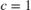, the behavior of the two degree-of-freedom PID controller is identical to a classical PID controller.

### Simulating with b = 1 and c = 1

Whenand, the behavior of the two degree-of-freedom PID controller is identical to a classical PID controller.The spikes in the control signal are caused by aggressive proportional and derivative response to the setpoint change, as the scope shows. Modifying the b and c weights can make this response less aggressive.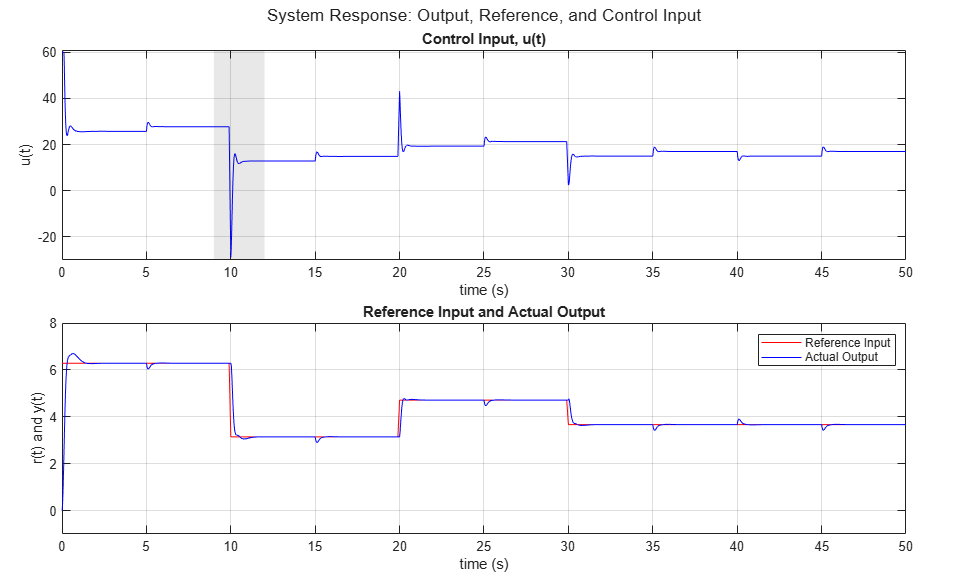### Simulating with_b_ = 0 and c = 0

In this case, the two degree-of-freedom PID controller is known as I-PD, where only the I action acts on the classical error signal, and the PD action acts only on the measured output.The simulation results show the absence of large transients in the control signal due to the sudden changes in the setpoint.

See  for more information on how to chose b and c.

If you have Simulink® Control Design™, you can use the PID tuner in to automatically tune all the PID Controller (2DOF) block gains (P, I, D, N, b, c).

 Åström, Karl J., and Tore Hägglund. Advanced PID Control. Research Triangle Park, NC: International Society of Automation, 2006.